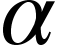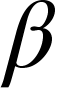Find the quadratic polynomial whose sum and product of roots are and -1 respectively.

# Find the quadratic polynomial whose sum and product of roots areand -1 respectively.

1. A
2. B
3. C
4. DFill Out the Form for Expert Academic Guidance!l

+91

Live ClassesBooksTest SeriesSelf Learning

Verify OTP Code (required)

### Solution:

The quadratic equation withandas roots is written asHere the sum of roots =Product of roots =-1## Related content

 Area of Square Area of Isosceles Triangle Pythagoras Theorem Triangle Formula Perimeter of Triangle Formula Area Formulae Volume of Cone Formula Matrices and Determinants_mathematics Critical Points Solved Examples Type of relations_mathematics+91

Live ClassesBooksTest SeriesSelf Learning

Verify OTP Code (required)